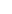This is a numeric average of given values. It is calculated by adding the value of each interval and dividing the total by the number of intervals covered by the SMA. For example, to calculate the 30-days SMA, we add the values of a given time of the last 30 days and then divide by 30. Although the daily value of a given time is the most common value used to calculate SMAs, the calculation may also be based on an average or daily average of the upper or lower values.

The SMA is a smoothing tool, which shows the basic trend of the market. This is one of the best ways to assess the strength of a long-term trend and the likelihood of a reversal. When the SMA is upward and the price exceeds it, the coin has an upward trend. Conversely, a downward average with the price at a lower level indicates a downward trend. A disadvantage is that it offers clues after the beginning of a new movement, an event or a trend, not before. Finally, one point of criticism is that it gives the same weight at all intervals. Some analysts believe that the latest price movements should be given greater weight.

SMA calculation example:

Suppose we are given the values of a given time (closing) of the last 6 days:

 Day 6 5 4 3 2 Today Closing price 5 3 2 4 7 9

To calculate the SMA of the last 6 days we add the closing values, i.e. 5 + 3 + 2 + 4 + 7 + 9 and divide the result by the number of days (6).
So: (5 + 3 + 2 + 4 + 7 + 9) / 6 = 30/6 = 5to signal pageSimple Moving Average (SMA) ❺❺❺ - average rating 5 from 5 (based on 201 user reviews)